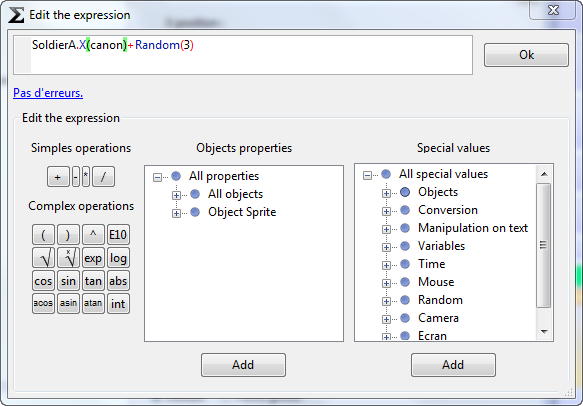# Expression Editor

The expression editor allows you to edit expressions in actions and conditions. An expression is a calculation using object properties like its position or angle, as shown at the top of the below image:## Expression structure

An expression is a mathematical calculation. It can be composed of numbers (e.g. 42), operators (e.g. 42 + 5), or functions (e.g. the random function: Random(5)).

A function is invoked by writing its name followed by parentheses. Parentheses optionally contain parameters to be sent to the function (e.g. Random(5)).

In order to invoke the function of an object, use the object name followed by a dot before the function (e.g. MyObject.X()).

## Help writing expressions

In the image above you can see a list of available operators and expressions: click to add one. You can also add properties in one of the two lists: click on Add, and fill out the parameters if necessary.

## Some examples and helpful functions

• You can convert text to numerical values using ToNumber. For example:

ToNumber(StrAt(“157”,Random(2)))

will give 1, 5, or 7 as a result.

• Random(x) will give a random number between 0 and x.